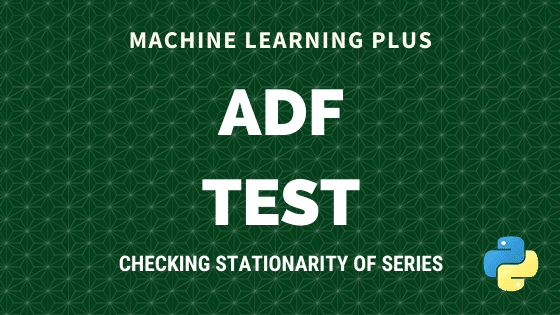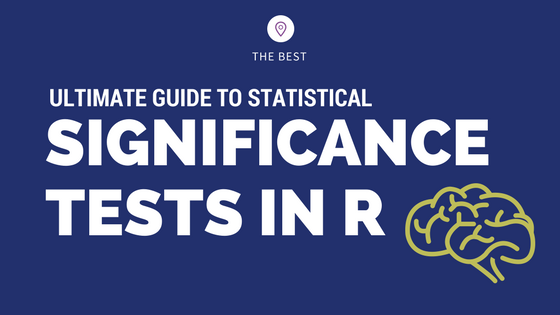# Significance Tests

## One Sample T Test – Clearly Explained with Examples | ML+

One sample T-Test tests if the given sample of observations could have been generated from a population with a specified mean. If it is found from the test that the means are statistically different, we infer that the sample is unlikely to have come from the population. For example: If you want to test a …Augmented Dickey Fuller test (ADF Test) is a common statistical test used to test whether a given Time series is stationary or not. It is one of the most commonly used statistical test when it comes to analyzing the stationary of a series. 1. Introduction In ARIMA time series forecasting, the first step is to …## What is P-Value? – Understanding the meaning, math and methods

P Value is a probability score that is used in statistical tests to establish the statistical significance of an observed effect. Though p-values are commonly used, the definition and meaning is often not very clear even to experienced Statisticians and Data Scientists. In this post I will attempt to explain the intuition behind p-value as …## How to implement common statistical significance tests and find the p value?

How to implement and interpret the commonly used statistical significance tests in R? Understand the purpose, when to use and how to interpret the test results and the p value. Correlation Test and Introduction to p value One Sample t-Test Wilcoxon Signed Rank Test Two Sample t-Test and Wilcoxon Rank Sum Test Shapiro Test Kolmogorov …Course Preview

## Machine Learning A-Z™: Hands-On Python & R In Data Science

### Free Sample Videos:#### Machine Learning A-Z™: Hands-On Python & R In Data Science#### Machine Learning A-Z™: Hands-On Python & R In Data Science#### Machine Learning A-Z™: Hands-On Python & R In Data Science#### Machine Learning A-Z™: Hands-On Python & R In Data Science